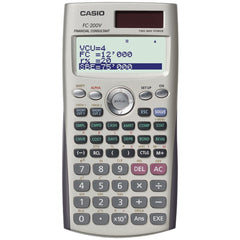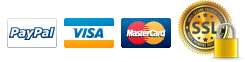# CASIO FC-200V Financial Calculator

##### Vendor: CASIO
4-line display; 10+2 digits; Simple & compound interest calculation; Dot matrix, investment appraisal (cash flow) & amortization; Converts between percentage interest rate & effective rate; Day calculation, depreciation & bond...

Available

\$59.994-line display; 10+2 digits; Simple & compound interest calculation; Dot matrix, investment appraisal (cash flow) & amortization; Converts between percentage interest rate & effective rate; Day calculation, depreciation & bond calculation; Break-even point calculation; Cost/sell/margin statistics; Slide-on hard case; Solar plus with battery backup;Panel Tool
Float header
Float topbar
Default Boxed Large Boxed Medium